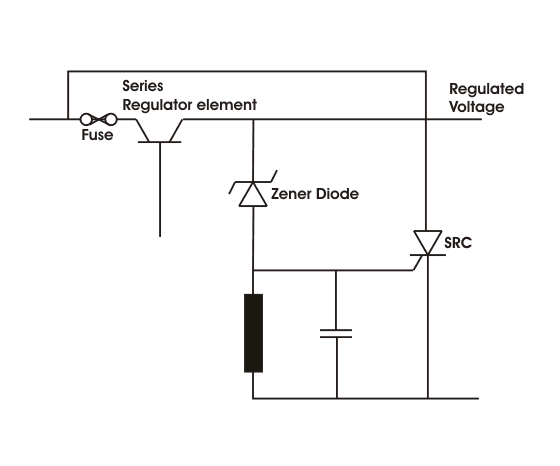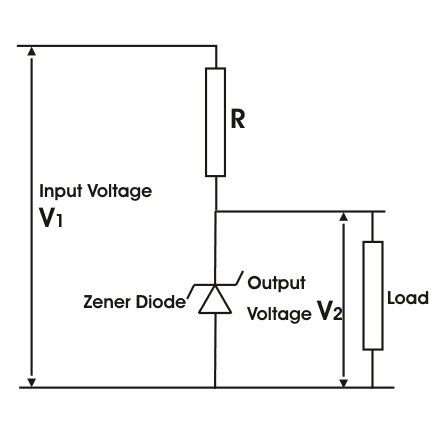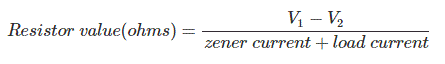# Applications of Zener Diode

We know what is Zener diode and what is the basic principle of operation of it. Now the question arises where this type of diodes are used. The main application of this type of diodes are as voltage regulator. Over voltage protector, as voltage reference. We will discussed what is the application of zener diode as voltage regulator, now we will discuss the other two points briefly. Overvoltage protection is done by using Zener diodes because there is current flowing through the diode after the reverse bias voltage exceeds a certain value. This circuit provides safety for the equipment connected to the terminals. Normally the current should not exceed conventional valve, but if due to any fault in the circuit the current exceeds the maximum allowable limit, the equipment of the system can be damaged permanently. A SCR is used, by it the output voltage is quickly cut down and a fuse blows which disconnects the input source power. The circuit arrangement is shown below for better understanding,Voltage references determine the constant supply of power, current or voltage as the zener voltage works if the supply of current is constant. That’s why to avoid unstable performance, zener diodes are used where voltage reference is required like ohmmeters, ammeters and voltmeters.

### Zener Diode as Voltage Regulator

The term regulator means which regulates or controls. Zener diode can work as a voltage regulator if it is introduced in a circuit. The output across the diode will be constant. It is driven by a current source. As we know if the voltage across the diode exceeds a certain value it would draw excessive current from the supply. The basic diagram of zener diode as voltage regulator is given below,To limit the current through the Zener diode series resistance R is introduced whose value can be chosen from the following equationThe above diagram is of a shunt regulator because the regulating element is parallel to the load. The Zener diode produce a stable reference voltage across the load which fulfills the criteria of the regulator.

Want To Learn Faster? 🎓
Get electrical articles delivered to your inbox every week.
No credit card required—it’s 100% free.